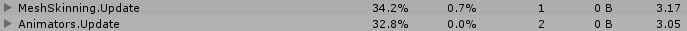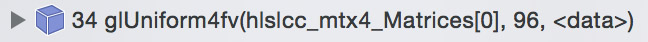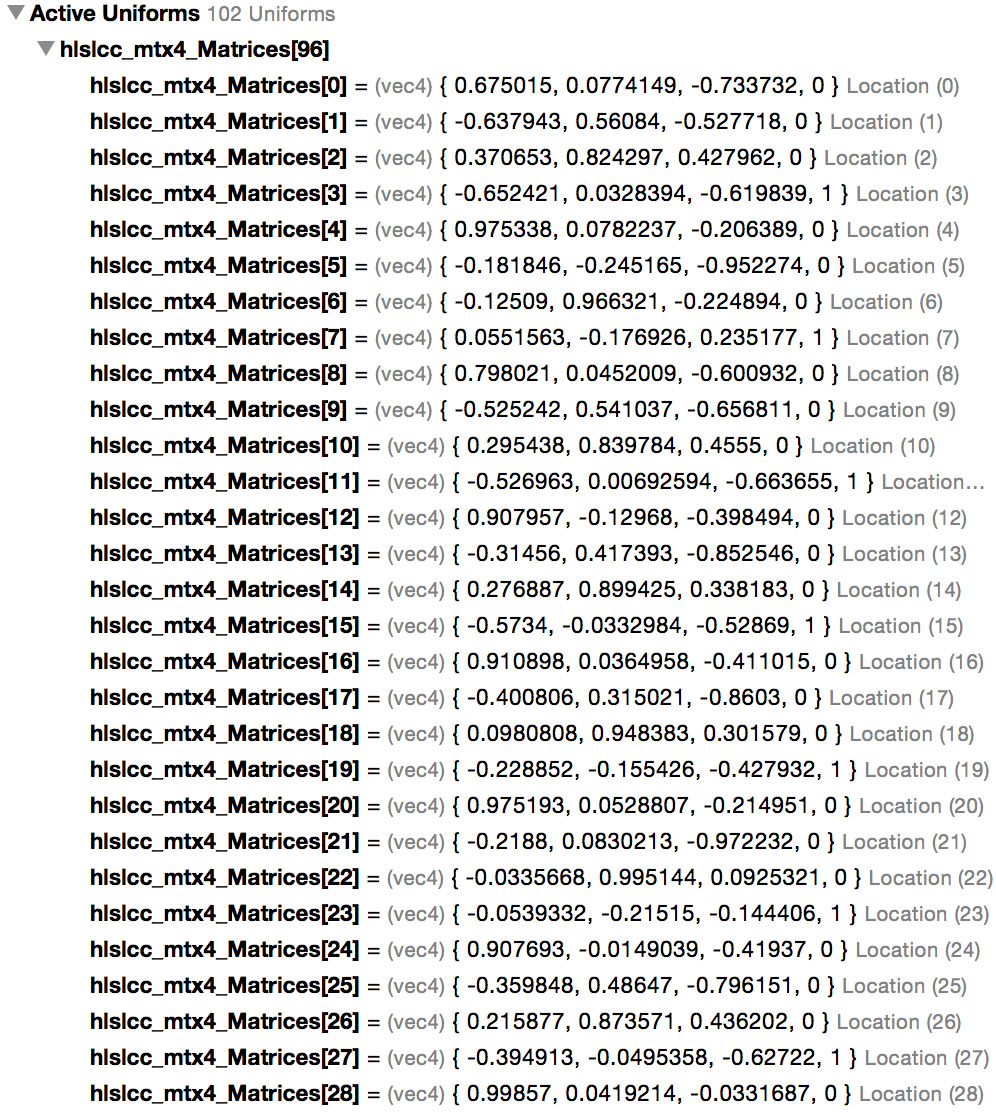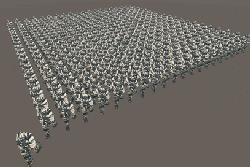# Jim's GameDev Blog# GPU Skinning

2016-11-272017-4-6:上面删除的那句话为我的理解错误，感谢知乎上燃野peter liu的指正，详见这篇文章后的评论。GLES3-Transform Feedback，DirectX 11-StreamOut。为此我又去仔细看了下细节，在开启 GPUSkinning 的时候，Unity 确实已经在 CPU 中进行了骨骼变换，而后将矩阵数组传递给 Shader，通过 Transform Feedback 后，将结果存储到 Buffer Object 中，这时 Buffer Object 中存储的顶点数据已经是蒙皮完成了，最后渲染模型的时候直接拿来用即可。下面这段 glsl 既是输出 Transform Feedback 的，也证明了这点。

#version 300 es

const int max_bone_count = 32;
const highp float max_bone_count_inv = 1.0 / float(max_bone_count);
const highp float half_texel = 0.5 * max_bone_count_inv;
in vec3 in_vertex;
in vec3 in_normal;
in vec4 in_tangent;
in ivec2 in_boneIndices;
in vec2  in_boneWeights;
out vec3 out_pos;
out vec3 out_normal;
out vec4 out_tangent;

uniform vec4 bones[max_bone_count*3];
#define GET_MATRIX(idx) mat4( bones[int(idx)*3 + 0], bones[int(idx)*3 + 1], bones[int(idx)*3 + 2], vec4(0.0, 0.0, 0.0, 1.0))

void main(void)
{
vec4 inpos = vec4(in_vertex.xyz, 1.0);
mat4 localToWorldMatrix = GET_MATRIX(in_boneIndices.x) * in_boneWeights;
if(in_boneWeights > 0.0)
localToWorldMatrix += GET_MATRIX(in_boneIndices.y) * in_boneWeights ;
out_pos = (inpos * localToWorldMatrix).xyz;
gl_Position = vec4(out_pos.xyz, 1.0);
out_normal = normalize( ( vec4(in_normal.xyz, 0.0) * localToWorldMatrix)).xyz;
out_tangent = vec4( normalize( ( vec4(in_tangent.xyz, 0.0) * localToWorldMatrix)).xyz, in_tangent.w);
}

• 将骨骼动画数据序列化到自定义的数据结构中。这么做是因为这样能完全摆脱 Animation 的束缚，并且可以做到 Optimize Game Objects（Unity 中一个功能，将骨骼的层级结构 GameObjects 完全去掉，减少开销），同时不丢失绑点。
• 在 CPU 中进行骨骼变换。
• 将骨骼变换的结果传递给 GPU，进行蒙皮。

### 提取骨骼动画数据Unity 中的 Animation 数据

EditorCurveBinding[] curvesBinding = AnimationUtility.GetCurveBindings(animClip);
foreach(var curveBinding in curvesBinding)
{
// 旋转
AnimationCurve curveRX = AnimationUtility.GetEditorCurve(animClip, curveBinding.path, curveBinding.type, "m_LocalRotation.x");
AnimationCurve curveRY = AnimationUtility.GetEditorCurve(animClip, curveBinding.path, curveBinding.type, "m_LocalRotation.y");
AnimationCurve curveRZ = AnimationUtility.GetEditorCurve(animClip, curveBinding.path, curveBinding.type, "m_LocalRotation.z");
AnimationCurve curveRW = AnimationUtility.GetEditorCurve(animClip, curveBinding.path, curveBinding.type, "m_LocalRotation.w");

// 位移
AnimationCurve curvePX = AnimationUtility.GetEditorCurve(animClip, curveBinding.path, curveBinding.type, "m_LocalPosition.x");
AnimationCurve curvePY = AnimationUtility.GetEditorCurve(animClip, curveBinding.path, curveBinding.type, "m_LocalPosition.y");
AnimationCurve curvePZ = AnimationUtility.GetEditorCurve(animClip, curveBinding.path, curveBinding.type, "m_LocalPosition.z");

// 不考虑缩放，假定所有骨骼的缩放都是 1

float curveRX_v = curveRX.Evaluate(second);
float curveRY_v = curveRY.Evaluate(second);
float curveRZ_v = curveRZ.Evaluate(second);
float curveRW_v = curveRW.Evaluate(second);

float curvePX_v = curvePX.Evaluate(second);
float curvePY_v = curvePY.Evaluate(second);
float curvePZ_v = curvePZ.Evaluate(second);

Vector3 translation = new Vector3(curvePX_v, curvePY_v, curvePZ_v);
Quaternion rotation = new Quaternion(curveRX_v, curveRY_v, curveRZ_v, curveRW_v);
NormalizeQuaternion(ref rotation);
Matrix4x4.TRS(translation, rotation, Vector3.one)
);
}

### 骨骼变换

private void Update()
{
// 更新 Walk 动作的所有骨骼变换
UpdateBoneAnimationMatrix("Walk", second);
second += Time.deltaTime;
}

private void UpdateBoneAnimationMatrix(string animName, float time)
{
// boneAnimation 是我们自定义的数据结构
// 其中存储了刚才从 AnimationCurve 中采样到的动画数据
GPUSkinning_BoneAnimation boneAnimation = GetBoneAnimation(animName);
int frameIndex = (int)(time * boneAnimation.fps) % (int)(boneAnimation.length * boneAnimation.fps);
// 获取当前播放的是哪一帧的动画数据
GPUSkinning_BoneAnimationFrame frame = boneAnimation.frames[frameIndex];

// 刷新所有的骨架动画矩阵
UpdateBoneTransformMatrix(bones[rootBoneIndex], Matrix4x4.identity, frame);
}

private void UpdateBoneTransformMatrix(GPUSkinning_Bone bone, Matrix4x4 parentMatrix, GPUSkinning_BoneAnimationFrame frame)
{
int index = BoneAnimationFrameIndexOf(frame, bone);
Matrix4x4 mat = parentMatrix * frame.matrices[index];
// 当前骨骼
bone.animationMatrix = mat * bone.bindpose;

// 继续递归子骨骼
GPUSkinning_Bone[] children = bone.children;
int numChildren = children.Length;
for(int i = 0; i < numChildren; ++i)
{
UpdateBoneTransformMatrix(children[i], mat, frame);
}
}

$M_{b1} = M_{root-trs} \ * \ ... \ * \ M_{b0-parent-parent-trs} \ * \ M_{b0-parent-trs} \ * \ M_{b0-trs} \ * \ M_{b1-trs} \ * \ M_{b1-bindpose}$

Bindpose 的作用是将模型空间中的顶点坐标变换到骨骼空间中（是骨骼矩阵的逆矩阵），然后应用当前骨骼的变换，沿着层级关系一层层的变换下去。

### 蒙皮

private void Update()
{
UpdateBoneAnimationMatrix("Walk", second);
Play();
second += Time.deltaTime;
}

private Matrix4x4[] matricesUniformBlock = null;
private void Play()
{
int numBones = bones.Length;
for(int i = 0; i < numBones; ++i)
{
matricesUniformBlock[i] = bones[i].animationMatrix;
}
// SetMatrixArray这是 Unity5.4 之后提供的新的 API
// 以前是不能直接传一个数组的，只能一个个元素单独传，效率很低
// 新的 API 减小了开销（看下图）
}// 模型确定为 24 个骨骼
// 不同的设备对常量寄存器存储的最大数据量都是有差别的，这一点需要注意
uniform float4x4 _Matrices;

struct appdata
{
float4 vertex : POSITION;
float2 uv : TEXCOORD0;
// tangent 存储了骨骼索引和权重
// tangent.x 第一根骨骼索引
// tangent.y 第一根骨骼权重
// tangent.z 第二根骨骼索引
// tangent.w 第二根骨骼权重
float4 tangent : TANGENT;
};

v2f vert (appdata v)
{
v2f o;

// 蒙皮
float4 pos =
mul(_Matrices[v.tangent.x], v.vertex) * v.tangent.y +
mul(_Matrices[v.tangent.z], v.vertex) * v.tangent.w;

// 注意，如果用到了 normal，也要像顶点一样经过蒙皮处理哦

o.vertex = mul(UNITY_MATRIX_MVP, pos);
o.uv = TRANSFORM_TEX(v.uv, _MainTex);
return o;
}

// Mesh.tangents 里预先存储了骨骼索引和权重
// tangent 里只容得下两个骨骼融合
Vector4[] tangents = new Vector4[mesh.vertexCount];
for(int i = 0; i < mesh.vertexCount; ++i)
{
BoneWeight boneWeight = mesh.boneWeights[i];
tangents[i].x = boneWeight.boneIndex0;
tangents[i].y = boneWeight.weight0;
tangents[i].z = boneWeight.boneIndex1;
tangents[i].w = boneWeight.weight1;
}
newMesh.tangents = tangents;